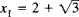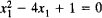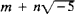# Algebraic Integer

Also found in: Wikipedia.

## algebraic integer

[¦al·jə¦brā·ik ′in·tə·jər]
(mathematics)
The root of a polynomial whose coefficients are integers and whose leading coefficient is equal to 1.
McGraw-Hill Dictionary of Scientific & Technical Terms, 6E, Copyright © 2003 by The McGraw-Hill Companies, Inc.
The following article is from The Great Soviet Encyclopedia (1979). It might be outdated or ideologically biased.

## Algebraic Integer

a number that is a root of an equation of the form xn + a1xn – 1 + · · · + an = 0, where a1,..., an are integers. For example,is an algebraic integer, since.

The theory of algebraic integers developed in the 1830’s and 1840’s as a result of the work of K. Jacobi, F. Eisenstein, and E. Kummer on reciprocity laws of higher degree, on Fermat’s theorem, and on the generalization of the arithmetic of complex integers.

The sum, difference, and product of algebraic integers are algebraic integers; that is, the set of algebraic integers forms a ring. The theory of the divisibility of algebraic integers, however, differs from the theory of the divisibility of ordinary integers. Algebraic integers of the form, where m and n are ordinary integers, are discussed in IDEAL.

References in periodicals archive ?
It was proved in  that if N C Fin([beta]) then [beta] is a Pisot number, that is, a real algebraic integer greater than 1 with all conjugates strictly inside the unit circle.
Algebraic integer (AI) encoding  has been proposed for addressing this problem.
By making use of Euclidean lengths of algebraic integral numbers in a related algebraic integer ring, we discuss the Frobenius expansions of algebraic numbers, theoretically and experimentally show that the multiplier in a scalar multiplication can be reduced and converted into a Frobenius expansion of length approximate to the field extension degree, and then propose an efficient scalar multiplication algorithm.
Let [theta] be an algebraic integer, and f (x) the minimal polynomial of 9 over Q.
The field F will be called norm-Euclidean if, for every pair ([alpha],[beta]) of algebraic integers in F with [beta] [not equal to] 0, there exists an algebraic integer [gamma] in F such that [absolute value of ([N.sub.F]([alpha] - [gamma][beta]))] < [absolute value of ([N.sub.F]([beta]))].
Then ([GAMMA], S) has the exponential growth rate [tau] = lim [sup.sub.k[right arrow][infinity]] [kth root of [a.sub.k]] which is a real algebraic integer bigger than 1 ().
Let [theta] be an algebraic integer in [k.sub.1] satisfying the following conditions:
Therefore [omega] > 1, the reciprocal of R, becomes a real algebraic integer whose conjugates have moduli less than or equal to the modulus of !.
Let n be a divisor of [q.sup.*], let [L.sub.n] be a subfield of K = Q([zeta]) with [[L.sub.n]: Q]= n and let [O.sub.n] be the algebraic integer ring of [L.sub.n].
Dubickas, Additive Hilbert's Theorem 90 in the ring of algebraic integers, Indag.
Rumely's introduction to this exposition on the Fekete-Szego theorem includes a sketch of the basic proof and contextualizes its development with the Robinson theorem on totally real algebraic integers in an interval and Cantor's extension with splitting conditions.
On the other side, algebraic integers lying in an extension field of the rationals are generated with the same linear polynomials.

Site: Follow: Share:
Open / Close